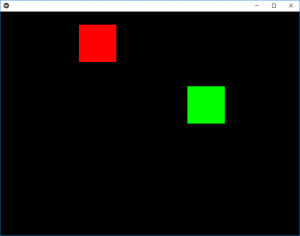# Python | Animation in Kivy using .kv file

Kivy is a platform independent GUI tool in Python. As it can be run on Android, IOS, linux and Windows etc. It is basically used to develop the Android application, but it does not mean that it can not be used on Desktops applications.

## Animation:

Animation and AnimationTransition are used to animate Widget properties. You must specify at least a property name and target value. To use Animation, follow these steps:

• Setup an Animation object
• Use the Animation object on a Widget

To use animation you must have to import:
from kivy.animation import Animation

```Basic Approaches:

1) import runTouchApp
2) import Builder
3) import Widget
4) import Animation
5) import Clock
6) import Window
7) import random
8) import listproperty
9) Build the .kv file
10) Create root class
11) Create the clock and anim react class used to animate the boxes
12) Run the App```

In the below example we are creating the two boxes (red and green) in which we are giving animations to the red box i.e when the App starts the red box is in its animated view but to clear the concepts i am providing the green box which on click provide random animations i.e it goes anywhere at random.
The program consists of two main classes first is clock class which is for the red box as we are declaring it animated and the green box animation is in anim class.

To provide the motion you must have to play with velocity.

Implementation of the Approach:

 `# work same as kivy.App used to run the App ` `from` `kivy.base ``import` `runTouchApp ` ` `  `# to use .kv file as a string we have to import it ` `from` `kivy.lang ``import` `Builder ` ` `  `# A Widget is the base building block of GUI interfaces in Kivy ` `from` `kivy.uix.widget ``import` `Widget ` ` `  `# The Clock object allows you to schedule a ` `# function call in the future ` `from` `kivy.clock ``import` `Clock ` ` `  `# Animation and AnimationTransition are ` `# used to animate Widget properties ` `from` `kivy.animation ``import` `Animation ` ` `  `# The Properties classes are used when ` `# you create an EventDispatcher. ` `from` `kivy.properties ``import` `ListProperty ` ` `  `# Core class for creating the default Kivy window.  ` `from` `kivy.core.window ``import` `Window ` ` `  `# As name suggest used when random thiungs required ` `from` `random ``import` `random ` ` `  ` `  `# load the kv file as string  ` `Builder.load_string(``''' ` `: ` ` `  `# Setting the position (initial) of boxes ` ` `  `    ``ClockRect: ` `        ``pos: 300, 300 ` `    ``AnimRect: ` `        ``pos: 500, 300 ` ` `  `# creation and animation of red box ` `: ` `    ``canvas: ` `        ``Color: ` `            ``rgba: 1, 0, 0, 1 ` `        ``Rectangle: ` `            ``pos: self.pos ` `            ``size: self.size ` ` `  `# creation and animation of red box ` `: ` `    ``canvas: ` `        ``Color: ` `            ``rgba: 0, 1, 0, 1 ` `        ``Rectangle: ` `            ``pos: self.pos ` `            ``size: self.size ` `'''``) ` ` `  ` `  `# Create the root class ` `class` `Root(Widget): ` `    ``pass` ` `  ` `  `# Create the clock class Then is when cliked ` `# how much time to animate ` `# the red colour block animation is created by it ` `class` `ClockRect(Widget): ` `    ``velocity ``=` `ListProperty([``10``, ``15``]) ` ` `  `    ``def` `__init__(``self``, ``*``*``kwargs): ` `        ``super``(ClockRect, ``self``).__init__(``*``*``kwargs) ` `        ``Clock.schedule_interval(``self``.update, ``1` `/` `60.``) ` ` `  `    ``def` `update(``self``, ``*``args): ` `        ``self``.x ``+``=` `self``.velocity[``0``] ` `        ``self``.y ``+``=` `self``.velocity[``1``] ` ` `  `        ``if` `self``.x < ``0` `or` `(``self``.x ``+` `self``.width) > Window.width: ` `            ``self``.velocity[``0``] ``*``=` `-``1` `        ``if` `self``.y < ``0` `or` `(``self``.y ``+` `self``.height) > Window.height: ` `            ``self``.velocity[``1``] ``*``=` `-``1` ` `  ` `  `# Create the Animation class ` `# And add animaton ` `# green colour box is animated through this class ` `class` `AnimRect(Widget): ` `     `  `    ``def` `anim_to_random_pos(``self``): ` `        ``Animation.cancel_all(``self``) ` `        ``random_x ``=` `random() ``*` `(Window.width ``-` `self``.width) ` `        ``random_y ``=` `random() ``*` `(Window.height ``-` `self``.height) ` ` `  `        ``anim ``=` `Animation(x ``=` `random_x, y ``=` `random_y, ` `                         ``duration ``=` `4``, ` `                         ``t ``=``'out_elastic'``) ` `        ``anim.start(``self``) ` ` `  `    ``def` `on_touch_down(``self``, touch): ` `        ``if` `self``.collide_point(``*``touch.pos): ` `            ``self``.anim_to_random_pos() ` ` `  `# run the App ` `runTouchApp(Root()) `

Output:Animated Output vedio:

My Personal Notes arrow_drop_upCheck out this Author's contributed articles.

If you like GeeksforGeeks and would like to contribute, you can also write an article using contribute.geeksforgeeks.org or mail your article to contribute@geeksforgeeks.org. See your article appearing on the GeeksforGeeks main page and help other Geeks.

Please Improve this article if you find anything incorrect by clicking on the "Improve Article" button below.

Article Tags :

Be the First to upvote.

Please write to us at contribute@geeksforgeeks.org to report any issue with the above content.